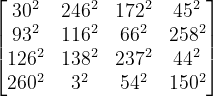# Magic square of squares

Allen William Johnson  discovered the following magic square whose entries are all squares.The following Python code verifies that this is a magic square.

    import numpy as np

M = np.array(
[[ 30**2, 246**2, 172**2,  45**2],
[ 93**2, 116**2,  66**2, 258**2],
[126**2, 138**2, 237**2,  44**2],
[260**2,   3**2,  54**2, 150**2]
])

def verify(M):

m, n = M.shape
assert(m == n)

c = sum(M[0, :])
semimagic = True
for i in range(m):
semimagic &= sum(M[i,:]) == c
semimagic &= sum(M[:,i]) == c

d1 = sum(M[i, i  ] for i in range(m))
d2 = sum(M[i,-i-1] for i in range(m))
magic = semimagic and (d1 == d2 == c)

if magic:
return "magic"
if semimagic:
return "semi-magic"
return "not magic"

print(verify(M))


## More magic square posts

 Allen William Johnson. Journal of Recreational Mathematics. 22 (1990), 38

## 5 thoughts on “Magic square of squares”

1. Now I’m curious if there exist any magic squares of the form […]^2, and if a hierarchy of squaring is possible.

2. James B.

Eat your heart out, Matt Parker!

3. Bob Lyons

FYI, the discoverer’s name is Allan W. Johnson, Jr.